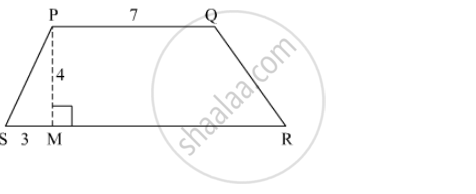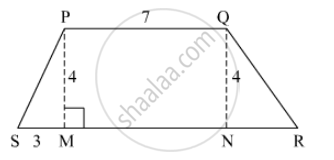Advertisement Remove all ads

# ☐ Pqrs is an Isosceles Trapezium L(Pq) = 7 Cm. Seg Pm ⊥ Seg Sr, L(Sm) = 3 Cm, Distance Between Two Parallel Sides is 4 Cm, Find the Area of ☐ Pqrs - Mathematics

Sum

☐ PQRS is an isosceles trapezium l(PQ) = 7 cm. seg PM ⊥ seg SR, l(SM) = 3 cm, Distance between two parallel sides is 4 cm, find the area of ☐ PQRSAdvertisement Remove all ads

#### SolutionDraw a perpendicular from Q to line MR. Where it meets the line MR name it point N.
MN = PQ = 7 cm
In Δ PMS,

PM² + SM² = PS²

⇒ 4² + 3² = PS²

⇒ PS² = 16 + 9 = 25

⇒ PS = 5cm

PQRS is an isosceles trapezium so, PS = QR = 5 cm
PM = QN = 4 cm
So, NR = SM = 3 cm
SR = SM + MN + NR = 3 + 7 + 3 = 13 cm
Area of trapezium PQRS = 1/2 xx (sum of parallel sides) x height

=1/2 xx (7 + 13) x 4

= 40 cm²

Is there an error in this question or solution?
Advertisement Remove all ads

#### APPEARS IN

Balbharati Mathematics 8th Standard Maharashtra State Board
Chapter 15 Area
Practice Set 15.3 | Q 3 | Page 99
Advertisement Remove all ads
Advertisement Remove all ads
Share
Notifications

View all notifications

Forgot password?